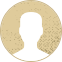Top Special Offer! Check discount

Get 13% off your first order - useTopStart13discount code now!

# Designing of Steel Beam

296 views 2 pages ~ 355 words
Get a Custom Essay Writer Just For You!

Experts in this subject field are ready to write an original essay following your instructions to the dot!

The section modulus of a particular shape is the cross sectional area of the structural member divided by the distance from the center of gravity to the farthest point of the section. It is also known as the flexural strength of the beam. Usually, it is a ratio of moment of inertia of a section about its centroid axis to the distance of extreme layer from the neutral axis. There are two types of section moduli, the elastic section modulus and the plastic section modulus. To calculate section modulus, we need to first calculate the maximum bending moments. A moment is a rotational force which occurs as a result of a perpendicular force being applied some distance away from the point.

Section modulus = Moment Of Inertia of the section / Distance from the axis to the extreme most layer

7kN                           65kN                                  6kN

10kN /m UDL

A     1.5m                                        6.5m                                                             2.0m         B

Taking moments at A clockwise moment = anticlockwise moments.

(1.5 X 7) + (65X4.75) + (6X6.5) = B(10)

10.5 + 308.75 + 39 = 10B

358.25 = 10B

35.82kN = B

Summation of upward forces = summation of downward forces.

35.82kN + A = 7kN + 65kN + 6kN

42.18kN   = A

Maximum Shear force = 42.18kN

Maximum bending Moment = 177.605 kN.m

Hence the required section modulus = Maximum bending moment / allowable normal stress

S    = 177.605 / 40

S   = 4.44m3

In our design we need to choose the beam with a section modulus of 4.44 therefore I chose the 152x152x23 designation because it meets the section modulus specifications and it has the lowest weight.

Part B

For us to select the most suitable beam we first need to calculate the elastic section modulus

Section modulus = Maximum bending moments / allowable normal stress for the steel beam

From the calculations, the maximum bending moment is equal to 1875kN.m

The yield stress given is 265Mpa

S = 1875kN.m / 265MPa

S    =   7.075m3

From the calculations we can deduce that the required section modulus for the column is 7.075m3.  The best choice for the column would be the 152x152x23 designation. The overall criteria used t select an appropriate beam is based on the mechanical design calculations. I chose the 152x152x23 designation because it meets the section modulus specifications and it has the lowest weight.

August 18, 2023
Subject area:
Number of pages

2

Number of words

355

41Rate:

5

Expertise Engineering
Verified writer

LuckyStrike has helped me with my English and grammar as I asked him for editing and proofreading tasks. When I need professional fixing of my papers, I contact my writer. A great writer who will make your writing perfect.

Hire Writer

This sample could have been used by your fellow student... Get your own unique essay on any topic and submit it by the deadline.

Eliminate the stress of Research and Writing!

Hire one of our experts to create a completely original paper even in 3 hours!